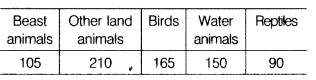# There are 720 creatures in a zoo as per list given below

(i)There are 720 creatures in a zoo as per list given belowRepresent the above data by a bar chart.
(ii) If we double the each type of creature, then whether bar chart of the new obtained data will be similar to the above data bar chart.

(i) Take zoo animals along X-axis and scale along X-axis is 2 units = 30 units.
Now, take the number of creatures along the Y-axis. All the bars should be of the same width and same space should be left between two consecutives bars.
These bars may be drawn as shown below(ii) Yes, the bar chart will be similar as above bar chart.